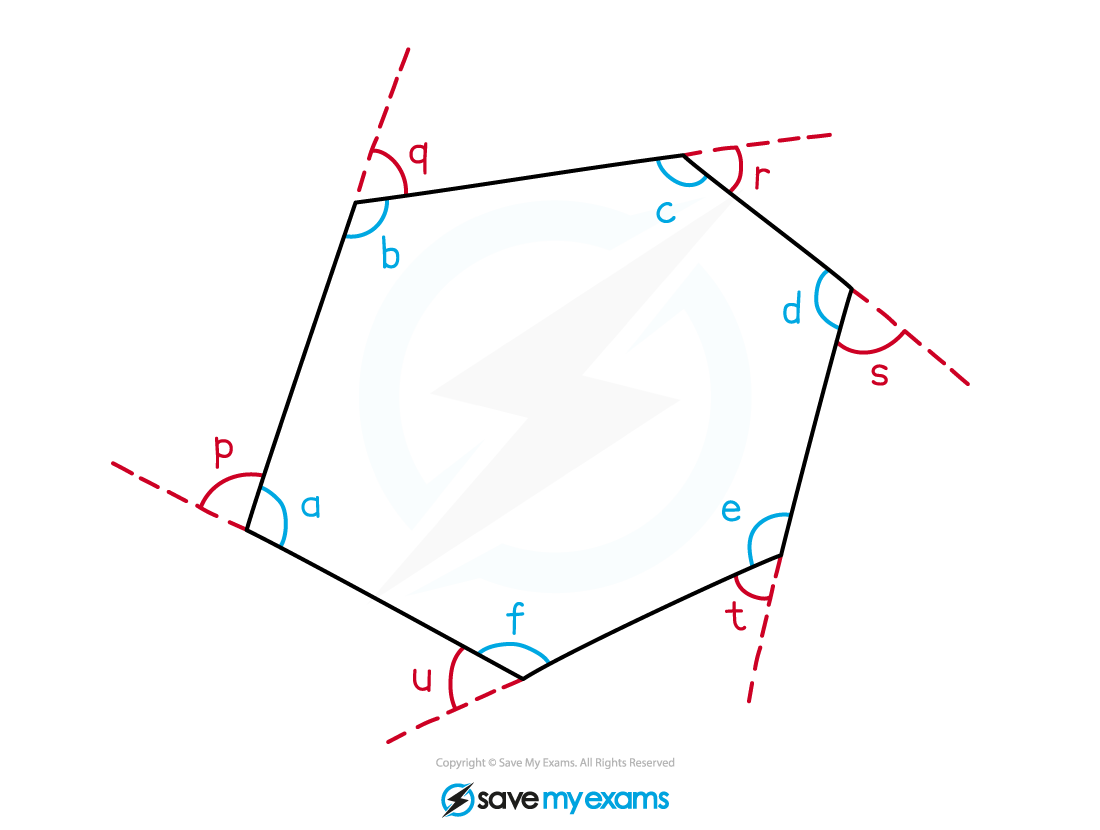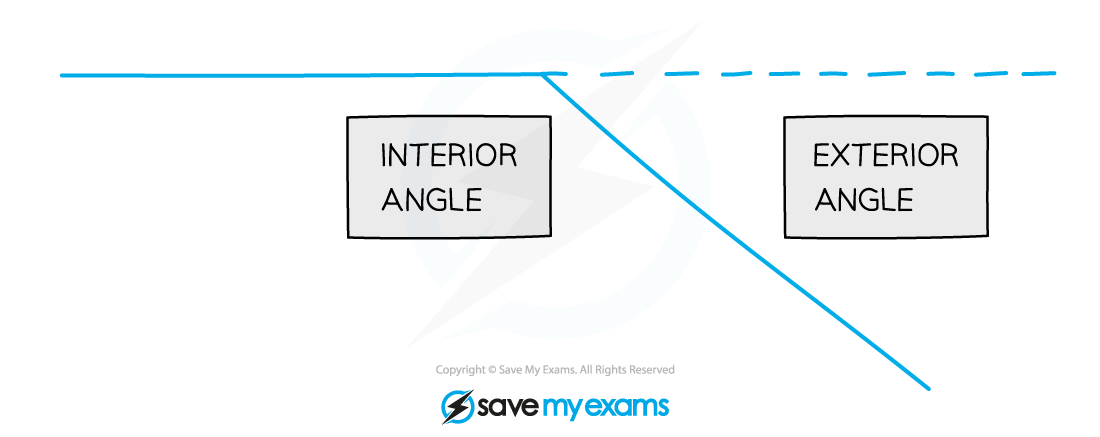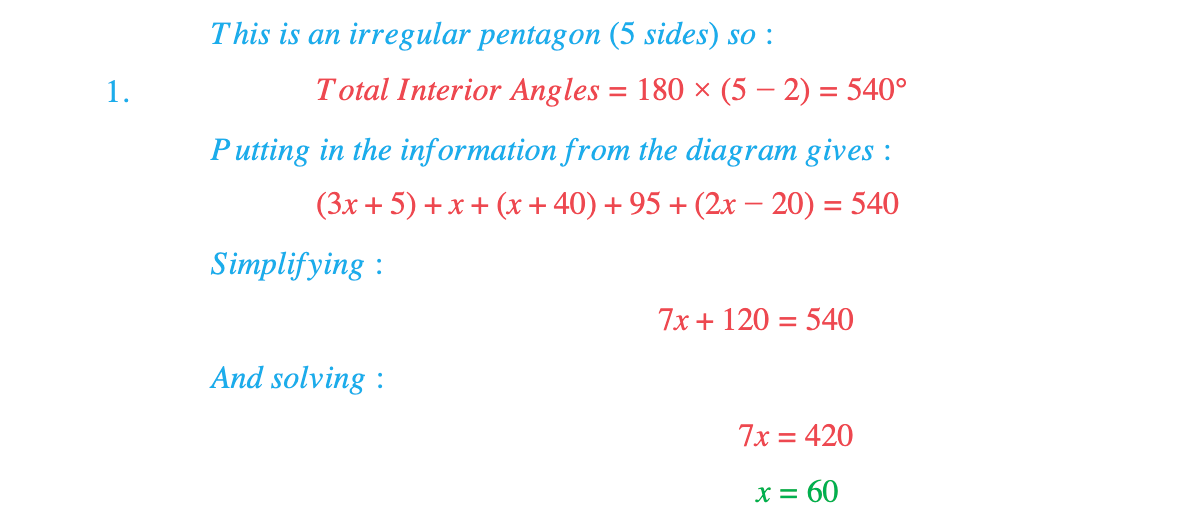# Edexcel IGCSE Maths 复习笔记 4.2.1 Angles in Polygons

Edexcel IGCSE Maths 复习笔记 4.2.1 Angles in Polygons

#### What is a polygon?

• polygon is a flat (plane) shape with n straight sidesFor example:

A triangle is a polygon with 3 sidesA quadrilateral polygon with 4 sides

A pentagon is a polygon with 5 sides

• In a regular polygon all the sides are the same length and all the angles are the same:

A regular polygon with 3 sides is an equilateral triangleA regular polygon with 4 sides is a square

#### Working with angles in polygons

1. TOTAL OF INTERIOR ANGLES = 180° × (n – 2)

(because the polygon can be split into n -2 triangles)2. TOTAL OF EXTERIOR ANGLES = 360°

(this exterior angles rule is the same for ANY number of sides!)eg For a hexagon (n=6):a + b + c + d + e + f = 180° × (6 – 2) = 720°

p + q + r + s + t + u = 360°For REGULAR Polygons:3. EXTERIOR ANGLE = 360 ÷ n4. INTERIOR ANGLE = 180 – Exterior Angle

#### Exam Tip

Make sure you know whether you are dealing with a regular or irregular polygon before you start a question.

#### Worked Example#### Worked Example